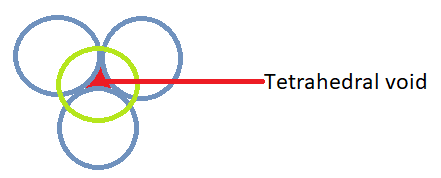QuestionAnswers

# Find the coordination number of $N{{a}^{+}}$ in $N{{a}_{2}}O$.

Hint: Disodium oxide has an anti-fluorite structure. The cations take up the void here. If we can find the type of void in the anti-fluorite structure, we can find the number of atoms which surround the sodium cation.

In disodium oxide, we have $N{{a}^{+}}\text{ and }{{\text{O}}^{2-}}$. As it has an anti-fluorite structure, the superoxide anion will lie in fcc and the sodium cation will take up the tetrahedral voids.Therefore, the correct answer is the coordination number of $N{{a}^{+}}$ in $N{{a}_{2}}O$ is 4.
Note: The alkali metals oxides with the structure ${{M}_{2}}O$ crystallize in fluorite or anti-fluorite structure. The Bravais lattice of fluorite or anti-fluorite structure is fcc i.e. face centered cubic. In such lattice, the lattice points are at every corner of the cube and also on each face centre.